# KSEEB Solutions for Class 7 Maths Chapter 8 Comparing Quantities Ex 8.2

Students can Download Class 7 Maths Chapter 8 Comparing Quantities Ex 8.2 Questions and Answers, Notes Pdf, KSEEB Solutions for Class 7 Maths helps you to revise the complete Karnataka State Board Syllabus and to clear all their doubts, score well in final exams.

## Karnataka State Syllabus Class 7 Maths Chapter 8 Comparing Quantities Ex 8.2

Question 1.
Convert the given fractional numbers to percents.
a)$\frac{1}{8}$
Solution:
Percent means per hundred. So multi-plied by 100.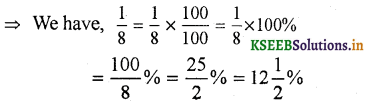b)$\frac{5}{4}$
Solution:c)$\frac{3}{40}$
Solution:d)$\frac{2}{7}$
Solution:Converting the fraction 9/20 as a decimal is very easy.

Question 2.
Convert the given decimal fractions to per cents.
a) 0.65
Solution:b) 2.1
Solution:c) 0.02
Solution:d) 12.35
Solution: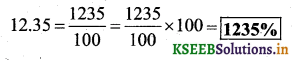Question 3.
Estimate what part of the figures is coloured and hence find the per cent which is coloured. (a) 0.65, (b) 2.1, (c) 0.02, (d) 12.35.Solution:
(i) $$\frac{1}{4}$$ of the figure is coloured.∴ 25% of the figure is coloured.

(ii) $$\frac{3}{5}$$ of the figure is coloured.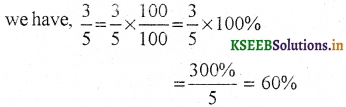∴ in the second figur e 60% Is coloured.

(iii) $$\frac{3}{8}$$ of the figure is coloured.∴ In the third figure 37.5 % is coloured.Question 4.
Find :
a) 15% of 250
Solution:b) 1% of 1 hour
Solution: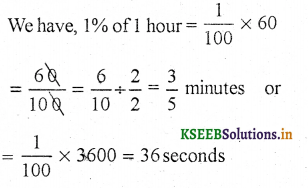∴ 1% of 1 hour is $$\frac{3}{5}$$ minutes or 36 seconds.

c) 20% of ₹ 2500
Solution:
We have, 20% of ₹ 2500
$$\frac{20}{100}$$ × 2500 = ₹ 500
∴ 20% of ₹ 2500 is ₹ 500.

d) 75% of  1 kg
Solution:
We have, 75% of 1 kg
$$\frac{75}{100}$$ × 1000 = 750 g
∴ 75% of 1 kg is 750 g.Question 5.
Find the whole quantity if
a) 5% of it is 600.
Solution:
Let the whole quantity be ‘m’
∴ 5% of m = 600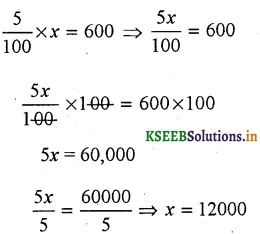∴ The whole quantity is 12,000.

b) 12% of its 1080.
Solution:
Let the whole quantity be ‘m’
∴ 12% of m = Rs. 1080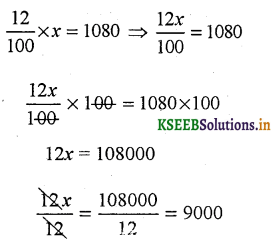∴ The whole amount be Rs. 9.000

c) 40 % of it is 500 km
Solution:
Let the whole quantity be ‘m’
∴ 40% of m = 500 km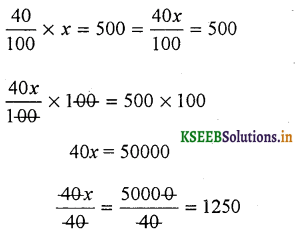∴ The whole amount is 1250 kmsd) 70% of it is 14 minutes
Solution:
Let the whole quantity be ‘m’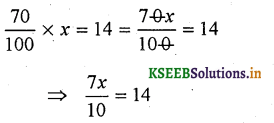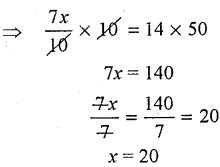∴ The whole amount is 20 minutes

e) 8% of it is 40 litres.
Solution:
Let the whole quantity be ‘m’∴ The whole amount be 500 litersQuestion 6.
Convert given per cents to decimal fractions and also to fractions in simplest forms:
Solution:
(a) 25%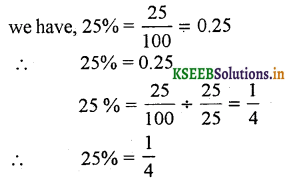(b) 150%(c) 20%(d) 5%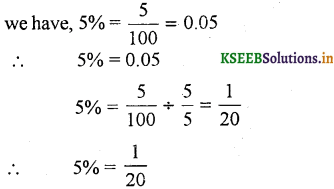Question 7.
In a city, 30% are females, 40% are males, and the remaining are children. What percent are children?
Solution:
females = 30%
males = 40%
= 100 – (30 + 40)
remaining are children = 100 – 70 = 30%
∴ Percentage of children = 30%Convert cgpa to percentage according mumbai university rule.

Question 8.
Out of 15,000 votes in a constituency, 60% voted. Find the percentage of voters who did not vote. Can you now find how many actually did not vote?
Solution:
Total no. of voters in a constituency = 15,000
Percentage of voted = 60%
∴ Percentage of voters who did not vote
= 100 – 60 = 40%
Actual number of voters who did not vote} = 40% of 15,000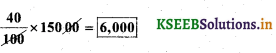6000 voters who did not vote.

Question 9.
Meeta saves ₹ 400 from her salary. If this is 10% of her salary. What is her salary?
Solution:
Let Meeta’s salary be ‘M’
10% of M is = Rs. 400
∴ M =?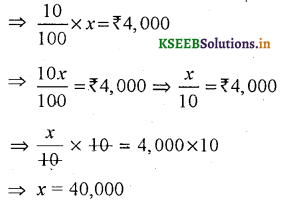∴ Her salary be Rs. 4,000/-

Question 10.
A local cricket team played 20 matches in one season. It won 25% of them. How many matches did they win?
Solution:
Let the total matches won by them be M 25% of 20 = M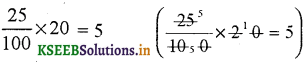∴ They win 5 matches.